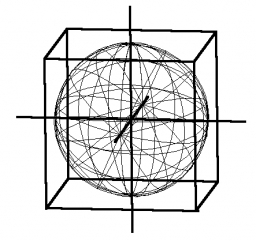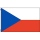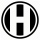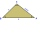# Cube into cylinder

If we dip a wooden cube into a barrel with a 40cm radius, the water will rise 10 cm. What is the size of the cube edge?

Result

a =  36.905 cm

#### Solution:

$r=40 \ \text{cm} \ \\ h=10 \ \text{cm} \ \\ \ \\ V=\pi \cdot \ r^2 \cdot \ h=3.1416 \cdot \ 40^2 \cdot \ 10 \doteq 50265.4825 \ \text{cm}^3 \ \\ \ \\ V=a^3 \ \\ \ \\ a=\sqrt{ V}=\sqrt{ 50265.4825 } \doteq 36.9054 \doteq 36.905 \ \text{cm}$Our examples were largely sent or created by pupils and students themselves. Therefore, we would be pleased if you could send us any errors you found, spelling mistakes, or rephasing the example. Thank you!

Leave us a comment of this math problem and its solution (i.e. if it is still somewhat unclear...):Be the first to comment!Tips to related online calculators
Do you want to convert length units?
Tip: Our volume units converter will help you with the conversion of volume units.

## Next similar math problems:

1. Oak trunkCalculate in tonnes the approximate weight of a cylindrical oak trunk with a diameter of 66 cm and a length of 4 m, knowing that the density of the wood was 800 kg/m³.
2. The hollow cylinderThe hollow cylinder has a height of 70 cm, an outer diameter of 180 cm and an inner diameter of 120 cm. What is the surface of the body, including the area inside the cavity?
3. Closed circuitIn a closed circuit, there is a voltage source with U1 = 12 V and with an internal resistance R1 = 0.2 Ω. The external resistance is R2 = 19.8 Ω. Determine the electric current and terminal voltage.
4. The basesThe bases of the isosceles trapezoid ABCD have lengths of 10 cm and 6 cm. Its arms form an angle α = 50˚ with a longer base. Calculate the circumference and content of the ABCD trapezoid.
5. Annulus from triangleCalculate the content of the area bounded by a circle circumscribed and a circle inscribed by a triangle with sides a = 25mm, b = 29mm, c = 36mm
6. A mapA map with a scale of 1: 5,000 shows a rectangular field with an area of 18 ha. The length of the field is three times its width. The area of the field on the map is 72 cm square. What is the actual length and width of the field?
7. Price reductionThe product is sold for 360 CZK and the sales profit is 30%. By what percentage the sales profit will be reduced if I reduce the price of the product by 10%?
8. Cllub flagThe children had the club flag sewn on. The red color covered 2/20 of the total area of the flag, the blue area a fifth, the yellow 19% of the total area. What% is the green area occupied?
9. The fourThe four pirates divided 65 coins to each other. They were sorted by age, the youngest receiving the least number of coins, each half more than the previous one. How many coins did the oldest pirate receive?
10. In aIn a triangle, the aspect ratio a: c is 3: 2 and a: b 5: 4. The perimeter of the triangle is 74cm. Calculate the lengths of the individual sides.
11. Five lettersHow many ways can five letters be arranged?
12. Job applicantsJob applicants: three-fourths of applicants had experience for a position. The number that did not have prior experience was 36. How many people applied for the job?
13. FlakesA circle was described on the square, and a semicircle above each side of the square was described. This created 4 "flakes". Which is bigger: the content of the central square or the content of four chips?
14. The trianglesThe triangles KLM and ABC are given, which are similar to each other. Calculate the lengths of the remaining sides of the triangle KLM, if the lengths of the sides are a = 7 b = 5.6 c = 4.9 k = 5
15. Large familyThe average age of all family members (children, mother, father, grandmother, grandfather) is 29 years. The average age of parents is 40 years, grandparents 66 years and all children are 5 years. How many children are there in this family?
16. Two trainsThe train runs at speed v1 = 72 km/h. The passenger, sitting in the train, observed that a train long l = 75m in 3 s passed on the other track in the opposite direction. Calculate the speed of this train.
17. Garden exchangeThe garden has the shape of a rectangular trapezoid, the bases of which have dimensions of 60 m and 30 m and a vertical arm of 40 m. The owner exchanged this garden for a parallelogram, the area of which is 7/9 of the area of a trapezoidal garden. What is